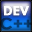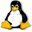Home Latest News Archive Tutorials C++ 2 3 4 Win32 Java OpenGL GLUT OpenGL ES 2 3 4 DirectX VB.NET HTML Photoshop 3dsmax Forums Projects Modelling Links About Us Search Recent Tutorials C / C++ Win32 OpenGL OpenGL ES GLUT## For Loop

Tutorials > C++ > For Loop

### Introduction

Another effective looping method is the for loop. This is probably the most frequently used of all the looping methods.

The for loop takes the form :

for ( initialization; logical expression; loop action ) { statements }

A description of each of the 3 sections inside parenthesis are shown below :

 Initialization : Actions to take before loop starts. Logical Expression : Condition that must hold for loop to run again. Loop Action : Actions to be taken each time the loop iterates (loops)

Contents of main.cpp :

```#include <iostream>
#include <stdlib.h>
#include <string>

using namespace std;

int main()
{
string data;
```

In the code segment below, nothing is entered into the 3 sections of the for loop. This basically causes no actions to be taken before the loop or after each iteration. It also specifies that there is no terminating condition. This will cause the for loop to continue running forever, resulting in an infinite loop.

How do we get out of the for loop. You may remember the break keyword from the switch statement tutorial. This keyword allows you to break out of any loop or case statement. We can therefore use it below to exit the loop when the user enters q.

```	for (;;)
{
cout << "Enter Data : ";
cin >> data;

if (data == "q")
break;
}

cout << endl;
```

The code segment below shows how a typical for loop is used.

Before the loop starts, an integer i is created and assigned the value of 0. The loop will continue running as long as i is less than 10. No action is taken at the end of each iteration.

The code will therefore print out all values from 0 to 9. 10 is not printed out as i is increased to 10 before the i < 10 is checked.

```	for (int i = 0; i < 10; )
cout << i++ << " ";

cout << endl;
```

The code below now shows how you can simplify the code above by utilizing the 3rd section of the for loop. An action can be specified to occur at the end of each loop iteration. Our action is the incrementing (increasing) of the i variable.

The code below will therefore print out exactly the same values as it did before, but the code will be easier to read and maintain.

10 is not printed out as the action is run before the check at the beginning of the loop.

```	for (int i = 0; i < 10; i++)
cout << i << " ";

cout << endl;
```

You may find it necessary to stop running a particular iteration of a loop and to start the loop again. The continue keyword can be useful here. It basically moves execution to the end of the loop skipping all statements between itself and the end of the loop.

In the code below, the printing out of the variable i will be skipped if the value is even. Any value % 2 is always 0 if it is a multiple of 2 ie. even. This could also be accomplished by using a simple if statement but you may find a situation where the continue keyword is the only option.

```	for (int i = 0; i < 10; i++)
{
if ((i % 2) == 0)
continue;

cout << i << " ";
}

cout << endl;

system("pause");

return 0;
}```

You should now understand how to use probably the most widely used loop of them all. In every useful program, you will find a number of for loops.

Please let me know of any comments you may have : Contact Me

 Source Files :< Tutorial 16 - Do-While Loop Tutorial 18 - Ternary Operator >